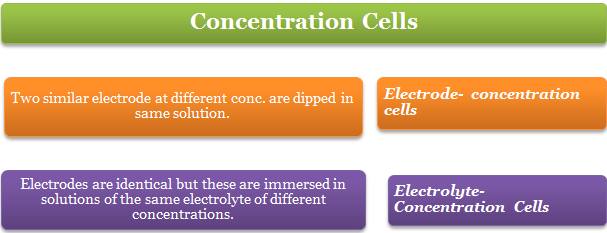×#### Thank you for registering.

One of our academic counsellors will contact you within 1 working day.

Click to Chat

1800-1023-196

+91-120-4616500

CART 0

• 0

MY CART (5)

Use Coupon: CART20 and get 20% off on all online Study Material

ITEM
DETAILS
MRP
DISCOUNT
FINAL PRICE
Total Price: Rs.

There are no items in this cart.
Continue ShoppingConcentration Cells

Table of Content

Electrode Concentration Cells

Electrolyte- Concentration Cells

Solved Examples

Related ResourcesThe cells in which EMF arises due to transfer of matter from one half cells to the other because of a difference in the concentration of species involved are known as concentration cells.

If two plates of the same metal are dipped separately into two solutions of the same electrolyte and are connected with a salt bridge, the whole arrangement is found to act as a galvanic cell. In general,

There are two types of concentration cells:Electrode Concentration Cells

Two similar electrode at different conc. are dipped in same solution e.g. two hydrogen electrodes at unequal pressure  are immersed in same solution of hydrogen ions.In these cells,

The potential difference is developed between two like electrodes at different concentrations dipped in the same solution of the electrolyte.

For example, two hydrogen electrodes at different has pressure in the same solution of hydrogen ions constitute a cell of this type.

(Pt,H2 (Pressure p1))/Anode |H+ | (H2 (Pressure p2)Pt)/Cathode
Pt; H2(p1) | H+ ions solution | H2(p2); Pt

(Pt,H2 (Pressure p1))/Anode |H+ | (H2 (Pressure p2)Pt)/Cathode

RHE

LHE

Overall

2H+ + 2e– →  H2(p2)

H2(p1)  →   2H+ + 2e–

H2 (p) →  H2 (p­2)

In the amalgam cells, two amalgams of the same metal at two different concentrations are interested in the same electrolyte solution.

Electrolyte- Concentration Cells

In these cells, electrodes are identical but these are immersed in solutions of the same electrolyte of different concentrations.

The source of electrical energy in the cell is the tendency of the electrolyte to diffuse from a solution of higher concentration to that of lower concentration.

With the expiry of time, the two concentrations tend to become equal. Thus, at the start the emf of the cell is maximum and it gradually falls to zero. Such a cell is repre­sented in the following manner:
(C2 is greater than C1).

M|Mn+(C1)||Mn+(C2)|M

Example: (Zn|Zn2+ (C1))/Anode || (Zn2+ (C2 )|Zn)/Cathode

(Zn|Zn2+ (C1))/Anode || (Zn2+ (C2 )|Zn)/Cathode

Anode

Cathode

Zn →  Zn2+(C1) + 2e

Zn2+(C2) + 2e– →  Zn(s)

The emf of the cell is given by the following expression at 25o C:

The concentration cells are used to determine the solubility of sparingly soluble salts, valency of the cation of the electrolyte and transition point of the two allotropic forms of a metal used as electrodes, etc.

For every concentration cell E° = 0.

Solved Examples

Question 1:

A cell contains two hydrogen electrodes. The negative electrode is in contact with a solution of 10-6 M hydrogen ions. The emf of the cell is 0.118volt at 25° C. Calculate the concentration of hydrogen ions at the positive electrode.

Solution:

The cell may be represented as

Pt|H2(1 atm)|H+||H+|H2(1 atm)|Pt

10-6 M   CM

Anode: H2 →  2H+ + 2e-

Cathode: 2H+ + 2e →  H2

Ecell = 0.0591/2 log([H+ ]cathode2)/[10-6 ]2

0.081 = (0.0591) log ([H+])/10-6

log[H+ ]cathode/10-6 =0.118/0.0591=2

[H+ ]cathode/10-6 = 102

[H+]cathode = 10-6 = 10-4 M

Question 2:

The emf of the cell Ag|Agl in 0.05 MK\Sol. NH4NO3|10.05 M AgNO3\Ag is 0.788 volt at 25°C. The activity coefficient of KI and silver nitrate in the above solution is 0.90 each. Calculate (i) the solubility product of Agl, and (ii) the solubility of Agl in pure water at 25°C.

Solution:

Ag+ ion concentration on AgN03 side = 0.9 × 0.5 = 0.045 M

Similarly I- ion concentration in 0.05 M KI solution  = 0.05 × 0.9 - 0.045 M

Ecell = 0.0591/1 log[Ag+ ](R.H.S.)/[Ag+ ](L.H.S.) = 0.0591 log 0.045/[Ag+ ](L.H.S.)

or

log 0.045/[Ag+ ](L.H.S.) = 0.788/0.0591 = 13.33

[Ag+]L.H.S. = 0.045/(2.138× 1013 ) = 2.105 × 10-15 M

Solubility product of Agl = [Ag+][I-]

= 2.105 × 10-15 × 0.045

= 9.427 × 10-17

Solubility of Agl = √(Solubility product of Agl)

= √(9.472×?10?^(-17) )

= 9.732 × 10-9 g mol L-1

= 9.732 × 10-9 × 143.5 g L-1

= 1.396 × 10-6 g L-1Question 1:

The observed emf of the cell

Pt|H2(1 atm)|H+(3×10-4 M)||H+(M1)|H2(1 atm)|Pt

is 0.154 V.

Vvalue of M1 of  cathodic solution is

a.  0.917

b. 5.421

c. 0.191

d. 7.321

Question 2:

What will be  the emf of the following cell at 25oC.

Pt H2|HCl|H2 Pt

2 atm        10 atm

a.  -5.0206 volt

b.  -3.0206 volt

c.  -1.0206 volt

d.  -0.0206 volt

Question 3:

For a concentration cell E° =

a. 0 V

b. 1 V

c. 2 V

d. 3 V

Question 4:

In concentration cells  EMF arises due to tansfer of

a. electrons,

b. charge

c. matter

d. protonsQ.1

Q.2

Q.3

Q.4

a

d

a

c

Related Resources

Refer to the Syllabus of Chemistry for IIT JEE

Look here for the  Past year Papers

To read more, Buy study materials of Electrochemistry comprising study notes, revision notes, video lectures, previous year solved questions etc. Also browse for more study materials on Chemistry here.### Course Features

• 731 Video Lectures
• Revision Notes
• Previous Year Papers
• Mind Map
• Study Planner
• NCERT Solutions
• Discussion Forum
• Test paper with Video Solution